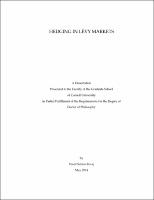## Hedging In Levy Markets##### Abstract
This dissertation examines the hedging process in L´ vy markets. It consists of e three self-contained chapters. In Chapter 1, we study some results from Malliavin calculus and their applications to the hedging problem. We set up a market with finite number of assets each driven by a L´ vy process and focus on the hedging problem. A selfe financing trading strategy is designed to perfectly hedge (if possible) a given contingent claim. If a perfect hedging is not possible, it is designed to approximately hedge a given contingent claim. In general, such a market is not complete and only a small subset of square integrable contingent claims can be hedged. For other claims, a minimum variance hedging strategy is given in literature in terms of Malliavin derivative of contingent claims and assets in the market. We review this result. Such a finite market can be completed by power jump assets. Malliavin derivative of each power jump asset is a polynomial and these polynomials are dense in square Lebesgue-integrable functions. In particular, Malliavin derivative of a contingent claim gives us a square integrable function and it can be approximated by Malliavin derivative of power jump assets. This result shows that enlarged market by infinitely many power jump assets gives us a market complete in the limit. In other words, a contingent claim in such a market (complete in the limit) can be either hedged perfectly or there exists a sequence of contingent claims, each can be hedged perfectly, such that mean squared hedging error goes to zero. Chapter 2 focuses on hedging in Heath-Jarrow-Morton model (HJM). We assume that forward rates are driven by a L´ vy process and summarize no- e arbitrage conditions in HJM framework. We develop necessary and sufficient conditions for perfect hedging of a contingent claim by a self-financing trading strategy. These conditions give us two integral equations: one eliminates Brownian motion related risk and another eliminates jump risk. The latter one takes the form of a Fredholm integral equation of the first kind and it does not have a solution for all possible square integrable contingent claims. Both integral equations have kernels in terms of forward rates and both equations are solved for Malliavin derivatives in different directions. First, we derive the solutions for the hedging problem when the market is driven by a pure jump process. Our analysis shows that under certain conditions, all power jump assets can be perfectly hedged. This result gives us sufficient conditions for a market which is complete in the limit. Further, we extend our results for a general L´ vy process. e As an application of our approach, we develop a hedging strategy for a variance swap which can be approximately hedged. We also obtain the Malliavin derivative of a caplet. Chapter 3 investigates hedging in exponential L´ vy markets. First, we ree view the call option pricing model that is derived on the basis of Fourier transform. Using Malliavin calculus, we develop the necessary and sufficient conditions for perfect hedging of a contingent claim by a self-financing trading strategy of call options. These conditions give us two integral equations: the first one relates to hedge of Brownian motion related risk and the other deals with hedging jump risk. First, we assume that market is driven by a pure jump process and jump sizes are bounded. In this case, we have only one integral equation which is related to jump risk. The integral equation is transformed into a con- volution type integral equation and a closed form solution for a dense subset of square integrable contingent claims is obtained in terms of Fourier transform and Malliavin calculus. We extend these results to general L´ vy process with e bounded jump sizes. Our approach can be used in practice to develop dynamic hedging strategies using call options. The above results have important implications for hedging in exponential L´ vy market: one key implication is that for e each possible jump size we need a call option with a strike value equal to jump size. We also show some results related to Asian options in exponential L´ vy e markets. Finally, we outline the major limitations of our approach.
2014-05-25
##### Keywords
Malliavin calculus; Levy processes; Hedging
##### Committee Chair
Jarrow, Robert A.
##### Committee Member
Gross, Leonard
Minca, Andreea C.
##### Degree Discipline
Operations Research
##### Degree Name
Ph. D., Operations Research
##### Degree Level
Doctor of Philosophy
##### Types
dissertation or thesis